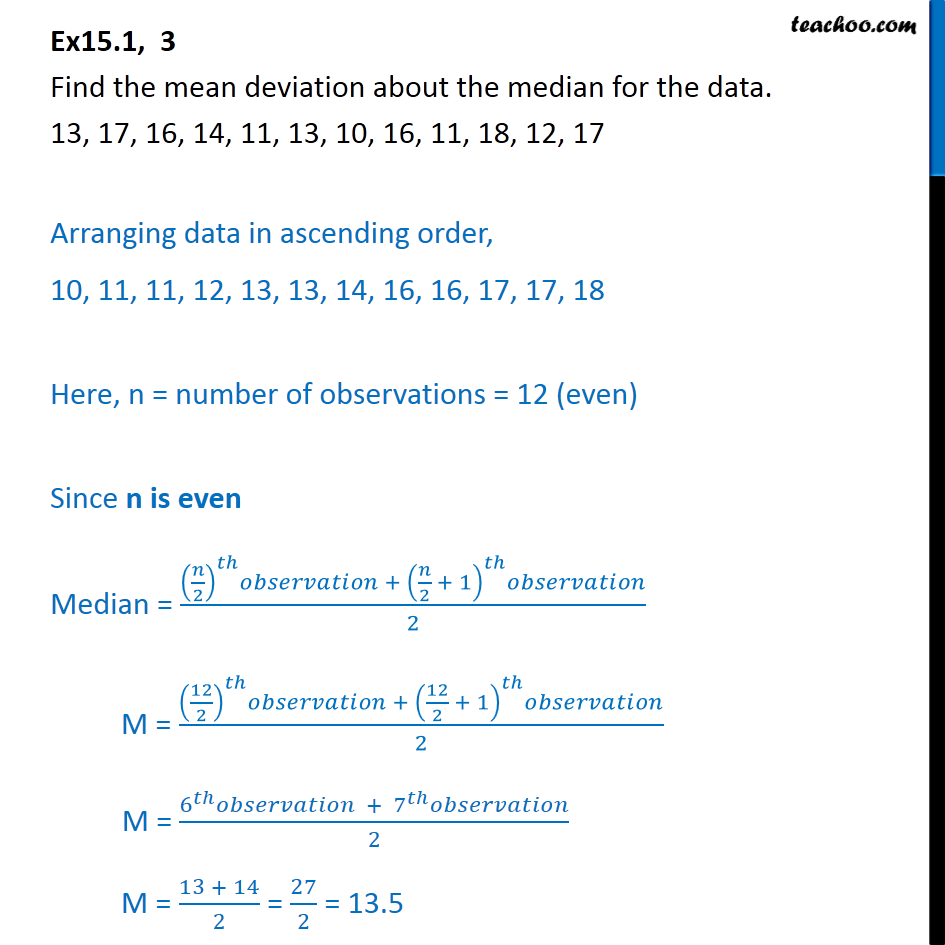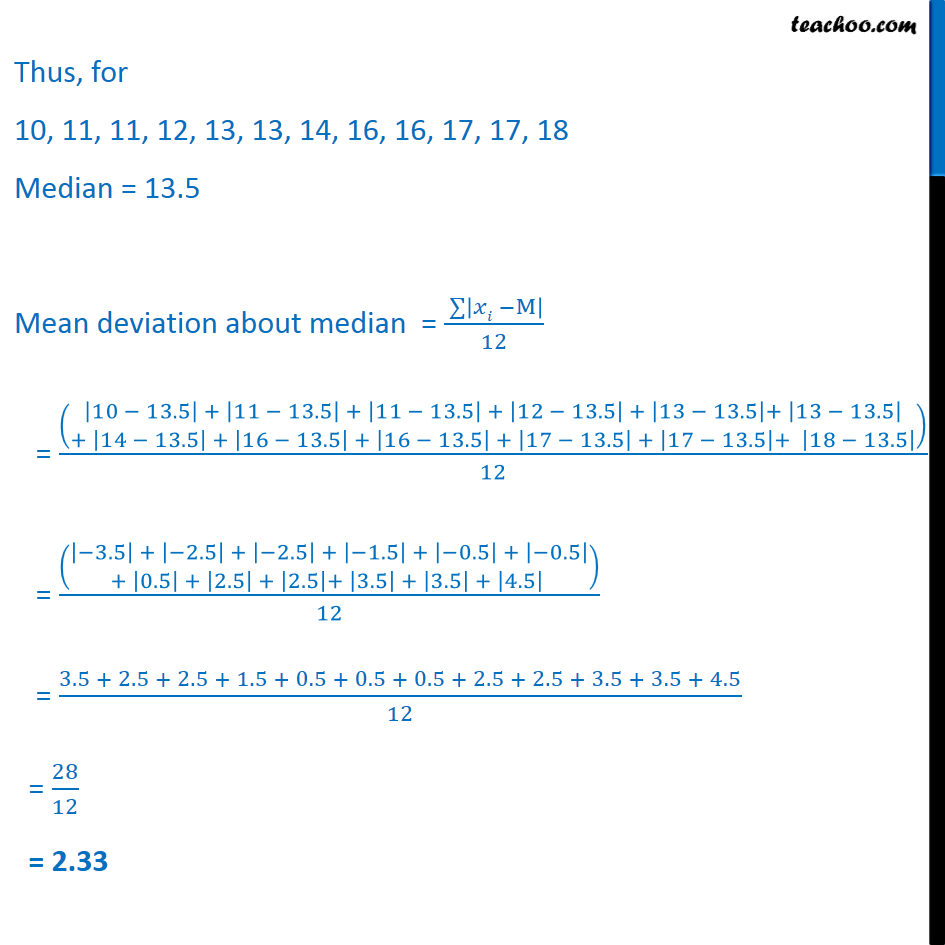Ex 13.1

Chapter 13 Class 11 Statistics
Serial order wiseLearn in your speed, with individual attention - Teachoo Maths 1-on-1 Class

### Transcript

Ex 13.1, 3 Find the mean deviation about the median for the data. 13, 17, 16, 14, 11, 13, 10, 16, 11, 18, 12, 17 Arranging data in ascending order, 10, 11, 11, 12, 13, 13, 14, 16, 16, 17, 17, 18 Here, n = number of observations = 12 (even) Since n is even Median = 𝑛﷮2﷯﷯﷮𝑡ℎ﷯𝑜𝑏𝑠𝑒𝑟𝑣𝑎𝑡𝑖𝑜𝑛 + 𝑛﷮2﷯ + 1﷯﷮𝑡ℎ﷯𝑜𝑏𝑠𝑒𝑟𝑣𝑎𝑡𝑖𝑜𝑛﷮2﷯ M = 12﷮2﷯﷯﷮𝑡ℎ﷯𝑜𝑏𝑠𝑒𝑟𝑣𝑎𝑡𝑖𝑜𝑛 + 12﷮2﷯ + 1﷯﷮𝑡ℎ﷯𝑜𝑏𝑠𝑒𝑟𝑣𝑎𝑡𝑖𝑜𝑛﷮2﷯ M = 6﷮𝑡ℎ﷯𝑜𝑏𝑠𝑒𝑟𝑣𝑎𝑡𝑖𝑜𝑛 + 7﷮𝑡ℎ﷯𝑜𝑏𝑠𝑒𝑟𝑣𝑎𝑡𝑖𝑜𝑛﷮2﷯ M = 13 + 14﷮2﷯ = 27﷮2﷯ = 13.5 Thus, for 10, 11, 11, 12, 13, 13, 14, 16, 16, 17, 17, 18 Median = 13.5 Mean deviation about median = ﷮﷮ 𝑥𝑖 −M﷯﷯﷮12﷯ = 10 − 13.5﷯ + 11 − 13.5﷯ + 11 − 13.5﷯ + 12 − 13.5﷯ + 13 − 13.5﷯+ 13 − 13.5﷯﷮+ 14 − 13.5﷯ + 16 − 13.5﷯ + 16 − 13.5﷯ + 17 − 13.5﷯ + 17 − 13.5﷯+ 18 − 13.5﷯﷯﷯﷮12﷯ = −3.5﷯ + −2.5﷯ + −2.5﷯ + −1.5﷯ + −0.5﷯ + −0.5﷯﷮+ 0.5﷯ + 2.5﷯ + 2.5﷯+ 3.5﷯ + 3.5﷯ + 4.5﷯ ﷯﷯﷮12﷯ = 3.5 + 2.5 + 2.5 + 1.5 + 0.5 + 0.5 + 0.5 + 2.5 + 2.5 + 3.5 + 3.5 + 4.5﷮12﷯ = 28﷮12﷯ = 2.33